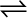7.2 What is Kc for the following equilibrium when the equilibrium concentration of each substance is: [SO2]= 0.60M, [O2] = 0.82M and [SO3] = 1.90M ?

2SO2(g) + O2(g)2SO3(g)

The equilibrium constant $\left({K}_{c}\right)$ for the given reaction is:
Hence, K for the equilibrium is .